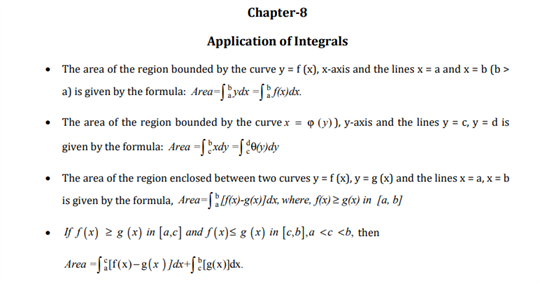# Maths Revision Notes for Class 12 Chapter 8 Application of Integrals.

For class 12 Maths, chapter 8 is titled as Application of Integrals whose revision notes can be found below. This chapter teaches students about the various applications that Integrals have. Some of the major topics taught here are the definition of definite integration along with the properties of definite integrals. With the help of these properties, students can gain proficiency in their understanding of the chapter and can improve their problem-solving skills as well. You can learn about other important concepts that are used while solving these problems, such as the limits of integration. The upper limit and the lower limit for a set of numbers form the limits of integration between the two numbers.

Hence, you can find the PDF of chapter 8 for CBSE class 12 Maths revision notes in the PDF below:You may also want to read:

 NCERT Related Articles NCERT Solutions for Class 12 NCERT Solutions for Class 11 NCERT Solutions for Class 10 NCERT Solutions for Class 12 Maths NCERT Solutions for Class 11 Maths NCERT Solutions for Class 10 Maths NCERT Solutions for Class 12 Physics NCERT Solutions for Class 11 Physics NCERT Solutions for Class 10 Science NCERT Solutions for Class 12 Chemistry NCERT Solutions for Class 11 Chemistry NCERT Solutions for Class 6 NCERT Solutions for Class 12 Biology NCERT Solutions for Class 11 Biology NCERT Solutions for Class 6 Maths NCERT Solutions for Class 9 NCERT Solutions for Class 8 NCERT Solutions for Class 6 Science NCERT Solutions for Class 9 Maths NCERT Solutions for Class 8 Maths NCERT Solutions for Class 4 NCERT Solutions for Class 9 Science NCERT Solutions for Class 8 Science NCERT Solutions for Class 4 Maths NCERT Solutions for Class 5 NCERT Solutions for Class 7 NCERT Solutions for Class 7 Maths NCERT Solutions for Class 4 Science NCERT Solutions for Class 5 Maths NCERT Solutions for Class 7 Science NCERT Solutions NCERT Books NCERT Solutions for Class 5 Science